## Course content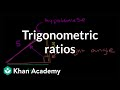0h09m

### Basic trigonometry | Basic trigonometry | Trigonometry | Khan Academy

Introduction to trigonometry

Practice this lesson yourself on KhanAcademy.org right now:

Trigonometry on Khan Academy: Big, fancy word, right? Don't be fooled. Looking at the prefix, tri-, you could probably assume that trigonometry ("trig" as it's sometimes called) has something to do with triangles. You would be right! Trig is the study of the properties of triangles. Why is it important? It's used in measuring precise distances, particularly in industries like satellite systems and sciences like astronomy. It's not only space, however. Trig is present in architecture and music, too. Now you may wonder...how is knowing the measurement and0h12m

### Basic trigonometry II | Basic trigonometry | Trigonometry | Khan Academy

A few more examples using SOH CAH TOA

Practice this lesson yourself on KhanAcademy.org right now:

Missed the previous lesson?

Trigonometry on Khan Academy: Big, fancy word, right? Don't be fooled. Looking at the prefix, tri-, you could probably assume that trigonometry ("trig" as it's sometimes called) has something to do with triangles. You would be right! Trig is the study of the properties of triangles. Why is it important? It's used in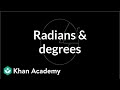0h09m

### Radian and degree | Unit circle definition of trig functions | Trigonometry | Khan Academy

Missed the previous lesson?

Trigonometry on Khan Academy: Big, fancy word, right? Don't be fooled. Looking at the prefix, tri-, you could probably assume that trigonometry ("trig" as it's sometimes called) has something to do with triangles. You would be right! Trig is the study of the properties of triangles. Why is it important? It's used in measuring precise distances, particularly in industries like satellite systems and sciences like astronomy. It's not only space, however. Trig is present in architecture and music, too. Now you ma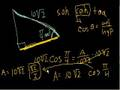0h10m

### Using Trig Functions

Using Trigonometric functions to solve the sides of a right triangle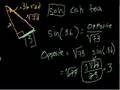0h08m

### Using Trig Functions Part II

A couple of more examples of using Trig functions to solve the sides of a triangle.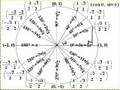0h09m

### The unit circle definition of trigonometric function

Using the unit circle to extend the SOH CAH TOA definition of the basic trigonometric functions.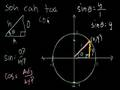0h10m

### Unit Circle Definition of Trig Functions

Using the unit circle to define the sine, cosine, and tangent functions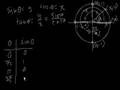0h07m

### Graph of the sine function

Using the unit circle definition of the sine function to make a graph of it.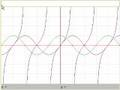0h10m

### Graphs of trig functions

Exploring the graphs of trig functions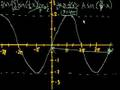0h09m

### Graphing trig functions

Analyzing the amplitude and periods of the sine and cosine functions.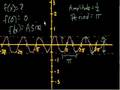0h05m

### More trig graphs

Determining the equations of trig functions by inspecting their graphs.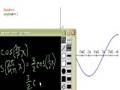0h09m

### Determining the equation of a trigonometric function

Determining the amplitude and period of sine and cosine functions.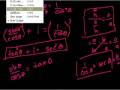0h09m

### Trigonometric Identities

Introduction to trigonometric identities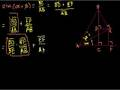0h09m

### Proof: sin(a+b) = (cos a)(sin b) + (sin a)(cos b)

Proof of the trig identity sin(a+b) = (cos a)(sin b) + (sin a)(cos b)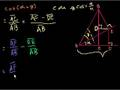0h09m

### Proof: cos(a+b) = (cos a)(cos b)-(sin a)(sin b)

Proof of the trig identity: cos(a+b) = (cos a)(cos b)-(sin a)(sin b)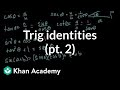0h09m

### Trig identities part 2 (part 4 if you watch the proofs) | Trigonometry | Khan Academy

More playing around with trig identities

Missed the previous lesson?

Trigonometry on Khan Academy: Big, fancy word, right? Don't be fooled. Looking at the prefix, tri-, you could probably assume that trigonometry ("trig" as it's sometimes called) has something to do with triangles. You would be right! Trig is the study of the properties of triangles. Why is it important? It's used in measuring precise distances, particularly in industries like satellite systems and sciences like astronomy. It's not only space, however. Trig is present in architecture and music, too. Now you may wonder...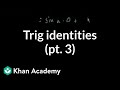0h08m

### Trig identities part 3 (part 5 if you watch the proofs) | Trigonometry | Khan Academy

Continuation of the playing around with trig identities

Missed the previous lesson?

Trigonometry on Khan Academy: Big, fancy word, right? Don't be fooled. Looking at the prefix, tri-, you could probably assume that trigonometry ("trig" as it's sometimes called) has something to do with triangles. You would be right! Trig is the study of the properties of triangles. Why is it important? It's used in measuring precise distances, particularly in industries like satellite systems and sciences like astronomy. It's not only space, however. Trig is present in architecture and music, too. Now you m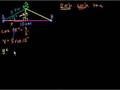0h09m

### Trigonometry word problems (part 1)

The first part of a problem when the captain of a ship goes off track.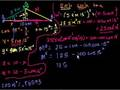0h02m

### Trigonometry word problems (part 2)

The second part of the problem of the off-track ship captain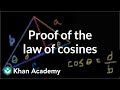0h09m

### Proof of the law of cosines | Trig identities and examples | Trigonometry | Khan Academy

Proof of the law of cosines to solve for a side of a triangle when 2 sides and an angle are known.

Practice this lesson yourself on KhanAcademy.org right now: